# Sound Waves

Waves are produced when elastic restoring forces and inertia cause vibrating atoms to oscillate, forming waves. Because atoms can vibrate in a variety of different patterns, waves can take many forms.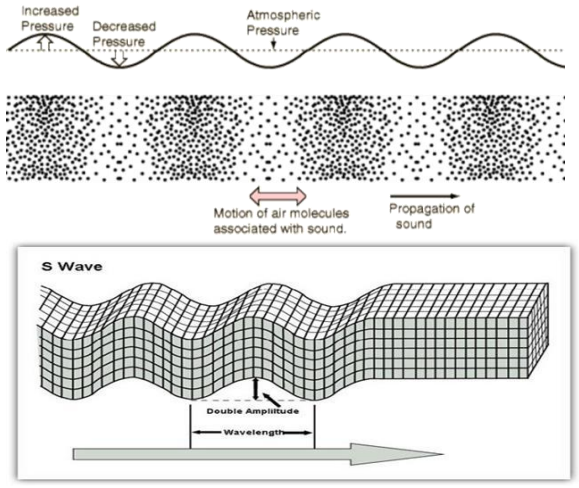Figure 7 and Figure 8: The motion of longitudinal waves (top)  and shear waves (bottom) .

Longitudinal waves occur when the particles oscillate in the same direction as the wave, and they can propagate through both solids and liquids. Longitudinal waves (see Figure 7) are also known as “density waves” because they result in particle density fluctuations, or “compression waves” because they involve compression and rarefaction (expansion). In shear waves, the particles oscillate perpendicular to the direction of the wave (see Figure 8). These waves are weaker than longitudinal waves and can only propagate through solids.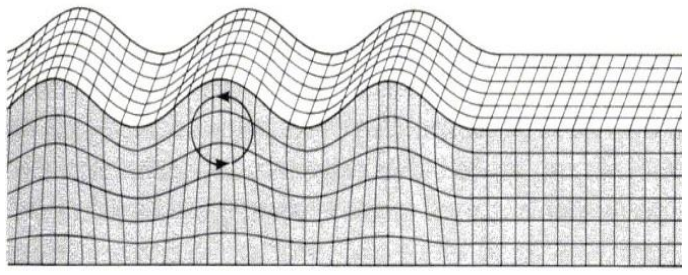Figure 9: The motion of Rayleigh waves .

Surface waves, or Rayleigh waves, contain particles that move in symmetrical elliptical orbits (see Figure 9). They travel along the surfaces of solid materials, and the elliptical motions of the particles decrease with increasing depth. Lastly, plate waves travel parallel to the surfaces of materials only a few wavelengths thick. They propagate through the entire material and can be either symmetric or asymmetric (see Figure 10). Since wave oscillations can be described by Hooke’s law, the speed of sound depends on the medium of propagation, not the amplitude of the wave. Precisely, the square of the wave’s velocity is equal to the elastic constant of the material divided by the density of the material .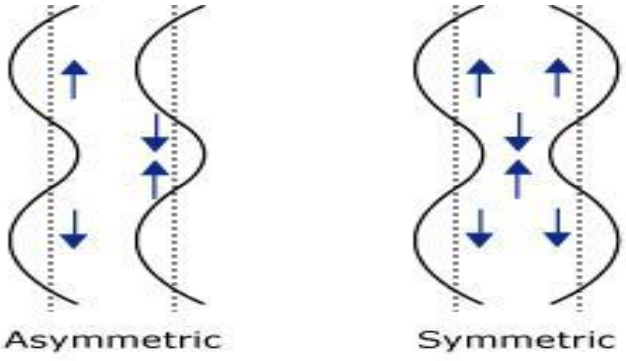Figure 10: Asymmetric and symmetric waves .

There are many ways sound waves can interact with other objects and each other. Reflection, the cause of echoes , occurs when a sound wave passes from one medium to another and some of the sound reflects rather than passing through the boundary . The amount of reflection depends on a medium’s acoustic impedance, a measure of a material’s resistance to sound propagation  which is equal to the product of the density of a material and the speed of sound through the material. Since the percent reflection (R) due to a change in medium is given by $R = \left( \frac{z_2 - z_1}{z_2 + z_1} \right)^2$ where $z_1$ and $z_2$ are the acoustic impedances of the two mediums, a greater acoustic impedance mismatch results in a larger amount of energy reflected . Reflection also depends on the angle of incidence, or the angle between the wave and the perpendicular to the surface of the medium. Maximum reflection occurs at a $90^\circ$ (normal) angle of incidence, and the percent reflection decreases as the angle of incidence becomes more oblique .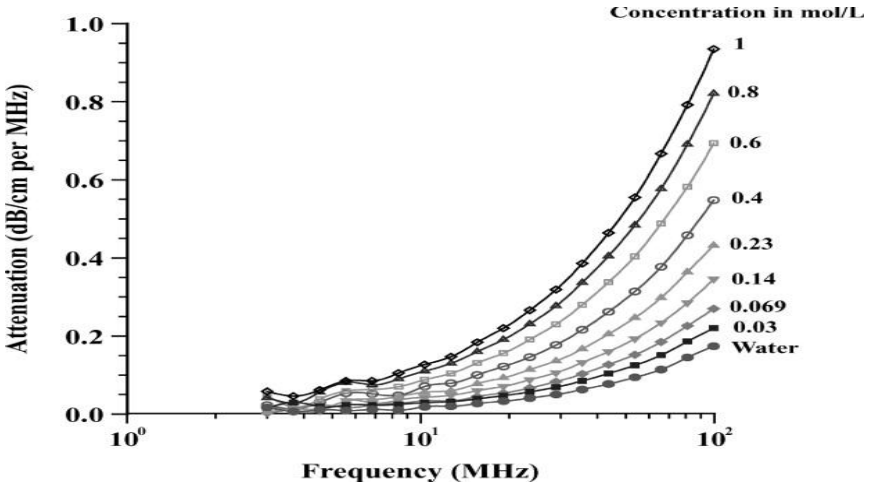Figure 11: Frequency vs. attenuation in water with varying concentrations of CuSO4 . As frequency increases, attenuation increases. Increasing density causes increasing attenuation as well.

Attenuation occurs when a sound wave’s intensity and amplitude decrease as it travels through a medium . 20% of the lost energy is attributed to scattering, a reflection of sound in other directions  caused when a sound wave contacts a rough or irregular surface. Absorption, or the conversion of sound energy to other forms of energy (usually heat), accounts for the remaining 80% of the lost energy. Since a wave’s frequency determines how fast its particles vibrate and particle vibrations release energy in the form of heat, attenuation increases as a wave’s frequency increases (see Figure 11). This explains why low-frequency sounds (bass) travel more effectively than high-frequency sounds .

Unlike attenuation, focusing occurs when refraction, the bending of sound waves, redirects the waves in a way that increases their intensity and amplitude. Refraction happens at the boundary of two mediums when there is an oblique incident angle and different speed of sound in the two mediums. The oblique incident angle allows one part of the wave to enter the second medium before the rest of the wave, and the different speed of sound in the second medium either accelerates or slows down the wave. The combination of these two effects causes the wave to change direction .

Other effects of sound include diffraction and interference. Diffraction is the tendency of sound waves to bend around small objects and spread out beyond small openings. Interference occurs when waves traveling in the same medium line up. When waves are in phase, their amplitudes add, resulting in constructive interference. When waves are out of phase, their amplitudes subtract, resulting in destructive interference .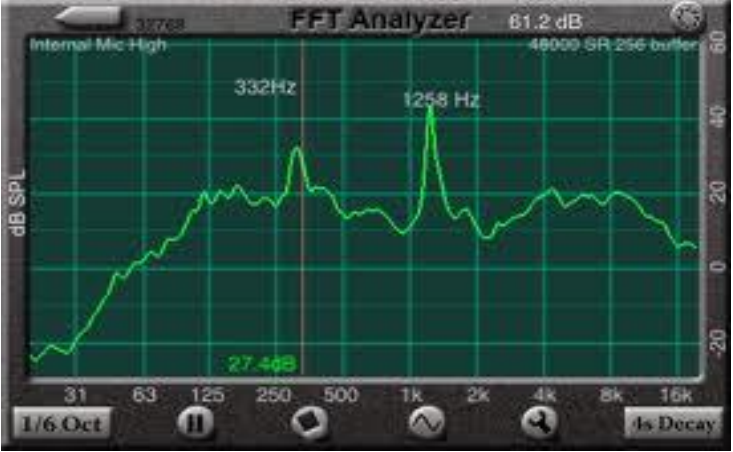Figure 12: A sample Fourier analysis . This sound wave has the most power at 332 Hz and 1258 Hz and has the least power at frequencies near 0 Hz.

Sounds can be measured and analyzed in a number of different ways. The intensity of a sound is its power per unit area , and it is determined by the sound’s amplitude. Intensity is often measured in decibels (dB), with the difference in decibels equal to $10 \log \left( \frac{V_2}{V_1} \right)$ dB, where $P_1$ and $P_2$ are the power measurements of two sounds and $P_1 < P_2.$ In terms of volts, the difference in decibels is equal to $20 \log \left( \frac{V_2}{V_1} \right)$ dB, provided $V_1 < V_2$ . Often, decibel measurements are taken relative to 1 Volt.

Using Fourier analysis, a sound can be analyzed by its frequency content. This is done by decomposing a sound waveform into sine and cosine waves with a Fast Fourier Transform and comparing the amplitudes of each harmonic  (see Figure 12).

## References

 Sound Waves in Air. N.d. HyperPhysics. Web. 5 Nov 2012. (link)

 S-Wave. N.d. ESG Solutions. Web. 7 Nov 2012. (link)

 Rayleigh wave motion process. 2004. SPICE Research Training Network and the University of Oslo. Web. 5 Nov 2012. (link)

 “Introduction to Ultrasonic Testing.” NDT Resource Center. N.p. Web. 22 Oct 2012. (link)

 N.d. NDT Resource Center. Web. 5 Nov 2012. (link)

 Baun, Jim. Physical Principles of General and Vascular Sonography. 24-34. Web. 25 Oct. 2012. (link)

 Dukhin, A. S., P. J. Goetz, et al. “Use of Ultrasound for Characterizing Dairy Products.” Journal of Dairy Science 88.4 (2005): 1320-1334. ScienceDirect. Database. 11 Dec 2012. (link)

 “Energy Threshold.” The Picasso Experiment. N.p., n.d

 “Sound Propagation.” HyperPhysics. Georgia State University. Web. 22 Oct 2012. (link)

 FFT. N.d. Studio Six Digital. Web. 7 Nov 2012. (link)

 “Sound Measurement.” HyperPhysics. Georgia State University. Web. 23 Oct 2012. (link)

 Zielinski, Tomasz G. Fundamentals of Piezoelectricity. Institute of Fundamental Technological Research. Web. 24 Oct 2012. (link)

 “What’s a Transducer?.” APC International, Ltd. Institute of Fundamental Technological Research. Web. 24 Oct 2012. (link)

 Wolfe, Joe. “dB: What is a decibel?.” Physclips. School of Physics UNSW, n. d. Web. 7 Nov. 2012. (link)

Tags: